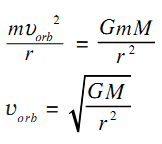# Orbital Velocity of Planets

If the orbital period of a planet is T and its distance from the Sun is r, then it covers a distance 2πr in time T. Its orbital velocity is

vorb = 2πr/T

There is another way also to calculate the orbital velocity. The centripetal force experienced by the planet must be supplied by the force of gravitation between the Sun and the planet. If M is the mass of the Sun, thenThe mass of the planet does not enter the above equation. The orbital velocity depends only on the distance from the Sun.